﻿ 树的最大离心距离和 On the Maximal Eccentric Distance Sum of Tree

Vol.06 No.04(2017), Article ID:21398,4 pages
10.12677/AAM.2017.64060

On the Maximal Eccentric Distance Sum of Tree

Xiaofei Wu, Xuegang Chen

Department of Mathematics, North China Electric Power University, Beijing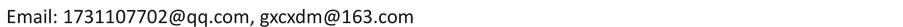Received: Jun. 24th, 2017; accepted: Jul. 15th, 2017; published: Jul. 18th, 2017ABSTRACT

Let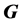be a connected graph. The eccentric distance sum of graphis defined as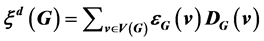, where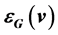is the eccentricity of the vertexand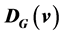is the sum of all distances from the vertex. In this paper, we characterize the tree with domination numberand the maximal eccentric distance sum. Some known results have been extended.

Keywords:Tree, Eccentricity, The Eccentric Distance Sum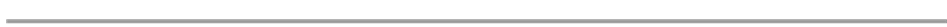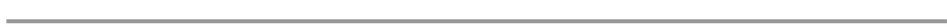1. 引言

2. 引理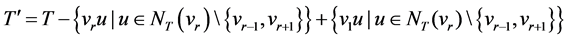，则有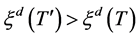是通过在路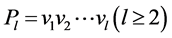的两个顶点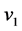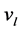上分别粘贴上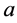个叶点和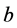个叶点得到的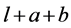阶树。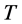是一个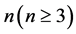阶树，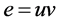的一条非悬挂边，设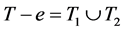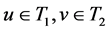，将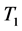中顶点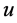中的顶点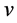粘在一起，并在顶点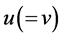上粘贴一个叶子，得到一个新树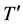，则称是对中的边做了一次边替换变换得到的。是一个阶树，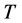的一条悬挂边，满足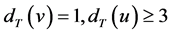，设边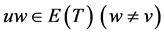，令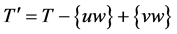。则称对边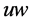的逆边替换变换得到的。Figure 1. Tree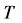and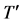3. 主要结论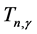表示控制数为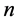阶树组成的集合。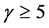时，对任意的阶树，设树中最长的路为，且设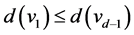。若从顶点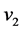到顶点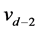中存在度数大于等于3的顶点，则令第一个度大于等于3的点为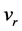，即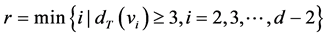.有最大的离心距离和。

On the Maximal Eccentric Distance Sum of Tree[J]. 应用数学进展, 2017, 06(04): 504-507. http://dx.doi.org/10.12677/AAM.2017.64060

1. 1. Geng, X.Y., Li, S.C. and Zhang, M. (2013) Extremal Values of the Eccentric Distance Sum of Trees. Discrete Applied Mathematics, 161, 2427-2439. https://doi.org/10.1016/j.dam.2013.05.023

2. 2. Hua, H.B., Xu, K.X. and Shu, W.N. (2011) A Short and Unified Proof of Yu et al’s Two Results on the Eccentric Distance Sum. Journal of Mathematical Analysis and Applications, 382, 364-366. https://doi.org/10.1016/j.jmaa.2011.04.054

3. 3. Miao, L.Y., Cao, Q.Q. and Cui, N. (2015) On the Extremal Values of the Eccentric Distance Sum of Trees. Discrete Applied Mathematics, 186, 199-206. https://doi.org/10.1016/j.dam.2015.01.042

4. 4. 朱晓颖, 逄世友. 控制数给定的树的最大离心距离和[J]. 山东大学学报(理学版), 2017, 52(2): 30-36.